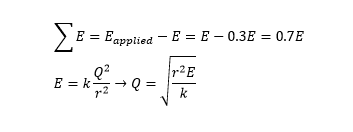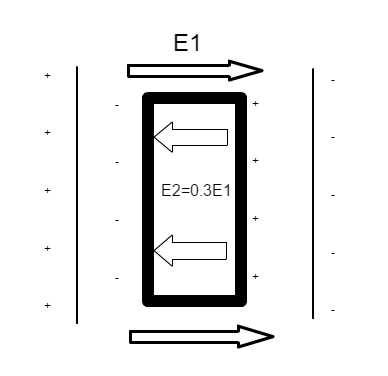# Simple capacitor problem

## Homework Statement

A capacitor connected to a battery initially holds a charge of +q on its positive plate and -q on its negative plate. The electric field between the plates is initially E. A dielectric material is then inserted that polarizes in such a way as to produce an electric field of -0.30E (where the - sign indicates that this field opposites that of E). Determine the new charge stored on the positive plate.

Eo-E=Ef
E=kq^2/r^2

## The Attempt at a Solution

I did my work on Word using the equation tool just to make the notation format easier to read.Is my logic correct here?

Any help would be greatly appreciated! :)

ehild
Homework Helper

## Homework Statement

A capacitor connected to a battery initially holds a charge of +q on its positive plate and -q on its negative plate. The electric field between the plates is initially E. A dielectric material is then inserted that polarizes in such a way as to produce an electric field of -0.30E (where the - sign indicates that this field opposites that of E). Determine the new charge stored on the positive plate.

Eo-E=Ef
E=kq^2/r^2

## The Attempt at a Solution

I did my work on Word using the equation tool just to make the notation format easier to read.Is my logic correct here?

Any help would be greatly appreciated! :)

The equation in red is the Coulomb force between two equal point charges. It is not electric field, and it is irrelevant to this problem.

The equation in red is the Coulomb force between two equal point charges. It is not electric field, and it is irrelevant to this problem.
Oh duh, it's just q from the source, not q^2

Could we solve it using the equation for capacitance?

ehild
Homework Helper
What i
Oh duh, it's just q from the source, not q^2

Could we solve it using the equation for capacitance?
What do you mean?

What i

What do you mean?

Well since it's capacitor maybe we can use C=Q/V=Q/Ed?

ehild
Homework Helper
Well since it's capacitor maybe we can use C=Q/V=Q/Ed?
That is correct. But the problem is not clear. Is the capacitor connected to the battery during the whole experiment?

That is correct. But the problem is not clear. Is the capacitor connected to the battery during the whole experiment?
Yes.

ehild
Homework Helper
Yes.
Does the electric field change between the plates then when the dielectric material is inserted?

Does the electric field change between the plates then when the dielectric material is inserted?

The field is the same between the empty space, but is different inside the dielectric, correct?

ehild
Homework Helper
No, the field is voltage over the distance between the plates, neither of them changes.

No, the field is voltage over the distance between the plates, neither of them changes.

Okay, so where can I go from there?

Can anyone else help me with this? =/

ehild
Homework Helper
Yes, the new charge is Q'=EεA. But you do not know ε.
The field polarizes the dielectric and dipole chains appear. The ends of the chains neutralize some of the surface charges. How much do they neutralize in this case? It was given, that the dipoles would make a field of 0.3 E, opposite to the original.

Yes, the new charge is Q'=EεA. But you do not know ε.
The field polarizes the dielectric and dipole chains appear. The ends of the chains neutralize some of the surface charges. How much do they neutralize in this case? It was given, that the dipoles would make a field of 0.3 E, opposite to the original.Does this image depict what you're describing correctly?

deleted

ehild
Homework Helper
Yes.

Yes.

Okay, so then would the new value for ε be the dielectric constant?
So κ=Eo/E = E/0.7E=1.4286?

ehild
Homework Helper
The new electric field is the same as the initial one was, U/d. See Post #10.

The new electric field is the same as the initial one was, U/d. See Post #10.

Okay, got it. So voltage is the same because of the presence of the battery and obviously separation does not change, thus field is constant. So how can I find the new charge if we don't know ε?

NascentOxygen
Staff Emeritus
Science Advisor
Your sketch in #16 suggests you are assuming that the added material occupies less than the full gap between the plates? However, this fraction doesn't seem to be specified.

ehild
Homework Helper
You know that E=E1-E2, as your picture shows. E2=0.3E. The new surface charge corresponds to the field E1, σ'=ε0E1. So what is σ' in terms of σ?

Your sketch in #16 suggests you are assuming that the added material occupies less than the full gap between the plates? However, this fraction doesn't seem to be specified.

That's true. I made the assumption because all of the examples that our professor has mentioned has been when there is some separation between the two. But the question never suggests that there is any.

You know that E=E1-E2, as your picture shows. E2=0.3E. The new surface charge corresponds to the field E1, σ'=ε0E1. So what is σ' in terms of σ?

σ=q/A

ehild
Homework Helper
I asked the relation between the new and the initial surface charges.